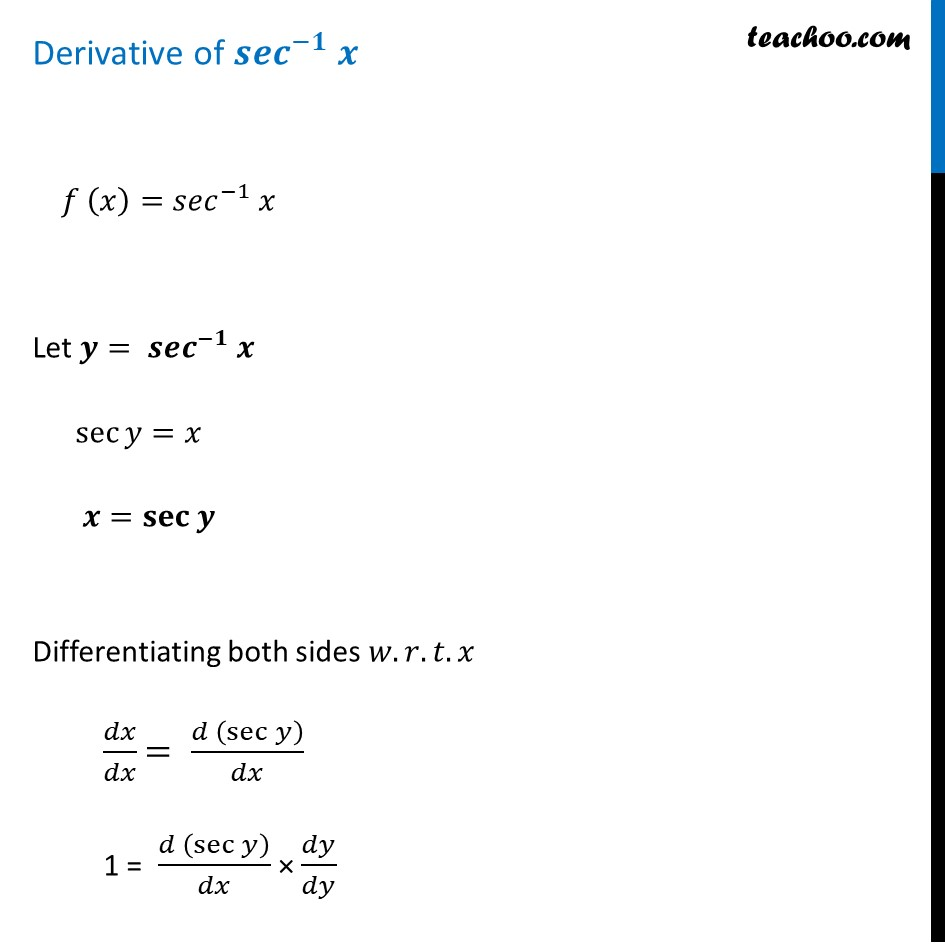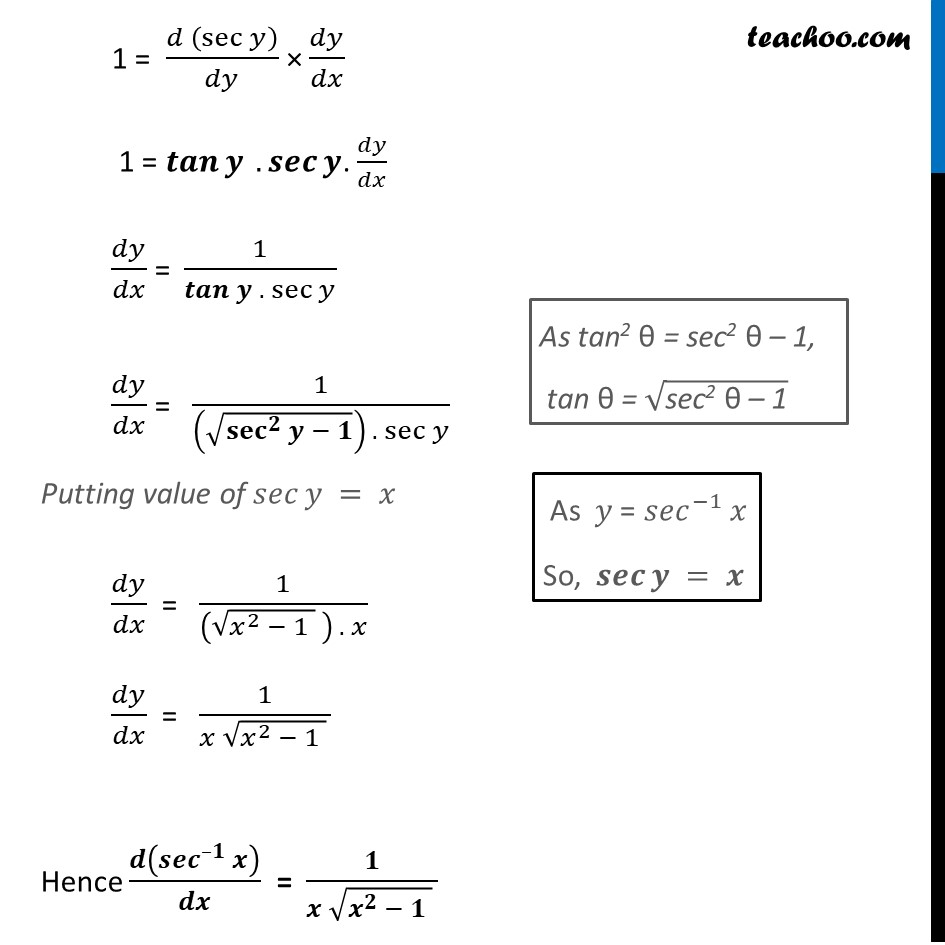Finding derivative of Inverse trigonometric functions

Chapter 5 Class 12 Continuity and Differentiability
Concept wiseLearn in your speed, with individual attention - Teachoo Maths 1-on-1 Class

### Transcript

Derivative of 〖𝒔𝒆𝒄〗^(−𝟏) 𝒙 𝑓 (𝑥)=〖𝑠𝑒𝑐〗^(−1) 𝑥 Let 𝒚= 〖𝒔𝒆𝒄〗^(−𝟏) 𝒙 sec⁡〖𝑦=𝑥〗 𝒙=𝐬𝐞𝐜⁡〖𝒚 〗 Differentiating both sides 𝑤.𝑟.𝑡.𝑥 𝑑𝑥/𝑑𝑥 = (𝑑 (sec⁡𝑦 ))/𝑑𝑥 1 = (𝑑 (sec⁡𝑦 ))/𝑑𝑥 × 𝑑𝑦/𝑑𝑦 1 = (𝑑 (sec⁡𝑦 ))/𝑑𝑦 × 𝑑𝑦/𝑑𝑥 1 = 𝒕𝒂𝒏⁡𝒚 .𝒔𝒆𝒄⁡𝒚. 𝑑𝑦/𝑑𝑥 𝑑𝑦/𝑑𝑥 = 1/(𝒕𝒂𝒏⁡𝒚 .〖 sec〗⁡𝑦 ) 𝑑𝑦/𝑑𝑥 = 1/((√(〖𝐬𝐞𝐜〗^𝟐⁡𝒚 − 𝟏)) .〖 sec〗⁡𝑦 ) Putting value of 𝑠𝑒𝑐⁡𝑦 = 𝑥 𝑑𝑦/𝑑𝑥 = 1/((√(𝑥^2 − 1 ) ) . 𝑥) 𝑑𝑦/𝑑𝑥 = 1/(𝑥 √(𝑥^2 − 1 ) ) Hence 𝒅(〖𝒔𝒆𝒄〗^(–𝟏) 𝒙)/𝒅𝒙 = 𝟏/(𝒙 √(𝒙^𝟐 − 𝟏 ) ) As tan2 θ = sec2 θ – 1, tan θ = √("sec2 θ – 1" ) As 𝑦 = 〖𝑠𝑒𝑐〗^(−1) 𝑥 So, 𝒔𝒆𝒄⁡𝒚 = 𝒙